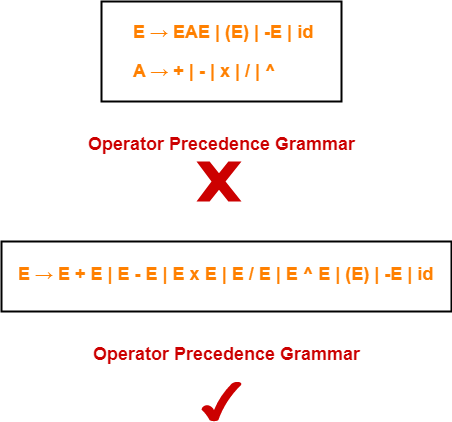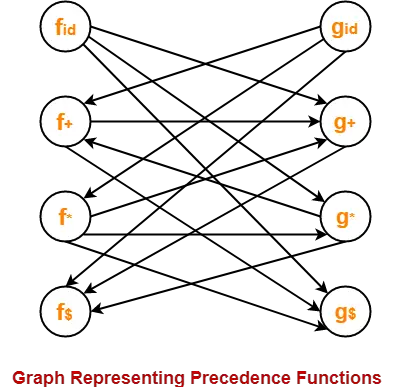## Operator Precedence Grammar-

 A grammar that satisfies the following 2 conditions is called as Operator Precedence Grammar–There exists no production rule which contains ε on its RHS.There exists no production rule which contains two non-terminals adjacent to each other on its RHS.

• It represents a small class of grammar.
• But it is an important class because of its widespread applications.

### Examples-## Operator Precedence Parser-

 A parser that reads and understand an operator precedence grammaris called as Operator Precedence Parser.

## Designing Operator Precedence Parser-

In operator precedence parsing,

• Firstly, we define precedence relations between every pair of terminal symbols.
• Secondly, we construct an operator precedence table.

## Defining Precedence Relations-

The precedence relations are defined using the following rules-

### Rule-01:

• If precedence of b is higher than precedence of a, then we define a < b
• If precedence of b is same as precedence of a, then we define a = b
• If precedence of b is lower than precedence of a, then we define a > b

### Rule-02:

• An identifier is always given the higher precedence than any other symbol.
• \$ symbol is always given the lowest precedence.

### Rule-03:

• If two operators have the same precedence, then we go by checking their associativity.

## Parsing A Given String-

The given input string is parsed using the following steps-

### Step-01:

Insert the following-

• \$ symbol at the beginning and ending of the input string.
• Precedence operator between every two symbols of the string by referring the operator precedence table.

### Step-02:

• Start scanning the string from LHS in the forward direction until > symbol is encountered.
• Keep a pointer on that location.

### Step-03:

• Start scanning the string from RHS in the backward direction until < symbol is encountered.
• Keep a pointer on that location.

### Step-04:

• Everything that lies in the middle of < and > forms the handle.
• Replace the handle with the head of the respective production.

### Step-05:

Keep repeating the cycle from Step-02 to Step-04 until the start symbol is reached.

The advantages of operator precedence parsing are-

• The implementation is very easy and simple.
• The parser is quite powerful for expressions in programming languages.

The disadvantages of operator precedence parsing are-

• The handling of tokens known to have two different precedence becomes difficult.
• Only small class of grammars can be parsed using this parser.

## Important Note-

• In practice, operator precedence table is not stored by the operator precedence parsers.
• This is because it occupies the large space.
• Instead, operator precedence parsers are implemented in a very unique style.
• They are implemented using operator precedence functions.

## Operator Precedence Functions-

 Precedence functions perform the mapping of terminal symbols to the integers.

• To decide the precedence relation between symbols, a numerical comparison is performed.
• It reduces the space complexity to a large extent.

## Problem-01:

Consider the following grammar-

E → EAE | id

A → + | x

Construct the operator precedence parser and parse the string id + id x id.

## Solution-

### Step-01:

We convert the given grammar into operator precedence grammar.

The equivalent operator precedence grammar is-

E → E + E | E x E | id

### Step-02:

The terminal symbols in the grammar are { id, + , x , \$ }

We construct the operator precedence table as-

 id + x \$ id > > > + < > < > x < > > > \$ < < <

### Parsing Given String-

Given string to be parsed is id + id x id.

We follow the following steps to parse the given string-

### Step-01:

We insert \$ symbol at both ends of the string as-

\$ id + id x id \$

We insert precedence operators between the string symbols as-

\$ < id > + < id > x < id > \$

### Step-02:

We scan and parse the string as-

\$ < id > + < id > x < id > \$

\$ E + < id > x < id > \$

\$ E + E x < id > \$

\$ E + E x E \$

\$ + x \$

\$ < + < x > \$

\$ < + > \$

\$ \$

## Problem-02:

Consider the following grammar-

S → ( L ) | a

L → L , S | S

Construct the operator precedence parser and parse the string ( a , ( a , a ) ).

## Solution-

The terminal symbols in the grammar are { ( , ) , a , , }

We construct the operator precedence table as-

 a ( ) , \$ a > > > > ( < > > > > ) < > > > > , < < > > > \$ < < < <

### Parsing Given String-

Given string to be parsed is ( a , ( a , a ) ).

We follow the following steps to parse the given string-

### Step-01:

We insert \$ symbol at both ends of the string as-

\$ ( a , ( a , a ) ) \$

We insert precedence operators between the string symbols as-

\$ < ( < a > , < ( < a > , < a > ) > ) > \$

### Step-02:

We scan and parse the string as-

\$ < ( < a > , < ( < a > , < a > ) > ) > \$

\$ < ( S , < ( < a > , < a > ) > ) > \$

\$ < ( S , < ( S , < a > ) > ) > \$

\$ < ( S , < ( S , S ) > ) > \$

\$ < ( S , < ( L , S ) > ) > \$

\$ < ( S , < ( L ) > ) > \$

\$ < ( S , S ) > \$

\$ < ( L , S ) > \$

\$ < ( L ) > \$

\$ < S > \$

\$ \$

## Problem-03:

Consider the following grammar-

E → E + E | E x E | id

1. Construct Operator Precedence Parser.
2. Find the Operator Precedence Functions.

## Solution-

The terminal symbols in the grammar are { + , x , id , \$ }

We construct the operator precedence table as-

 g → f ↓ id + x \$ id > > > + < > < > x < > > > \$ < < <

#### Operator Precedence Table

The graph representing the precedence functions is-Here, the longest paths are-

• fid  → gx → f+ → g+ → f\$
• gid  → fx → gx → f+ → g+ → f\$

The resulting precedence functions are-

 + x id \$ f 2 4 4 0 g 1 3 5 0

To gain better understanding about Operator Precedence Parsing,

Watch this Video Lecture